CD4560 is a 4-bit Natural Binary Coded Decimal (NBCD) Adder. It belongs to the CD4000 series of integrated circuits which is a CMOS based logic family. 74LS83 IC is also a BCD adder but belongs to a TTL logic-based family. CMOS logics dissipate lower power and they have standardized symmetrical outputs. The IC CD4560 adds two 4-bit numbers in natural binary coded decimal format and gives result of sum and output carry in NBCD. All inputs and outputs are active high. In case of no Carry in, the carry input for the least significant digit connects with Vss.

## CD4560 Pinout Diagram

CD4560 4-bit adder IC consists of sixteen pins. This is a pinout diagram.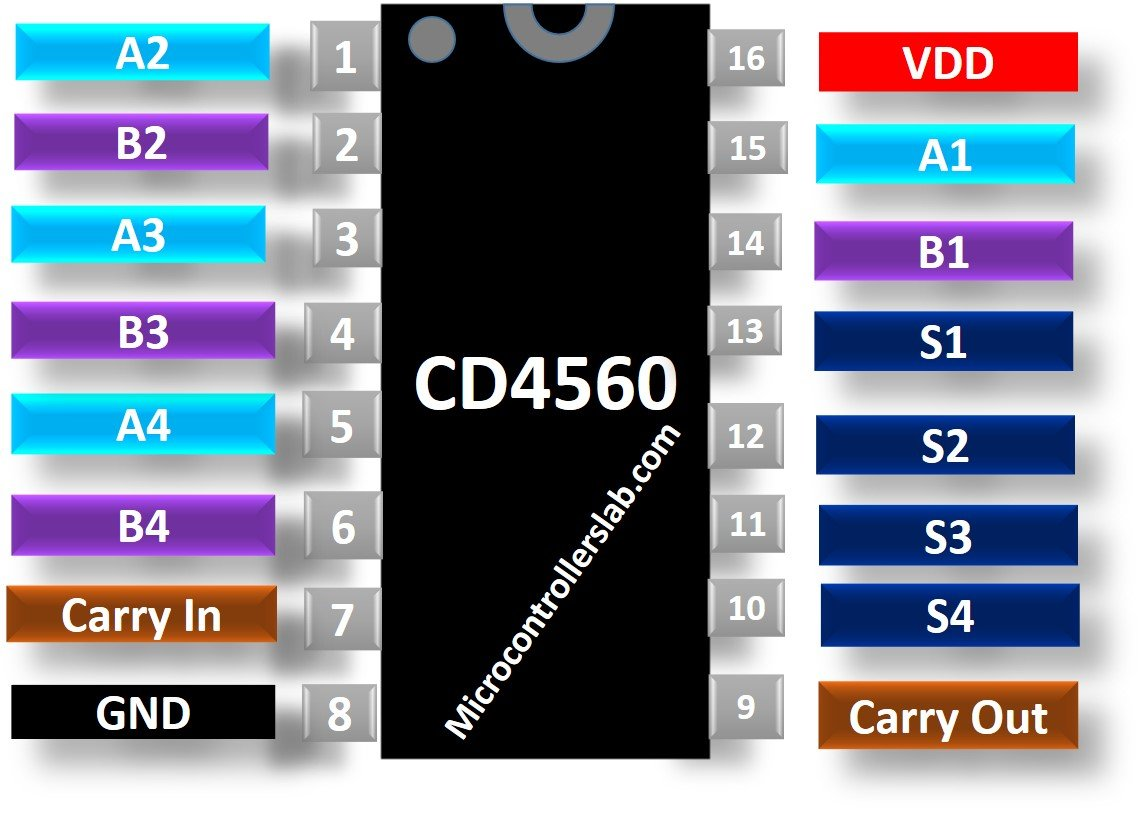### Pin Configuration Details

As we mentioned earlier, this IC has 16 pins in total and each having different functions. Nine pine are input pins such as A1-A4, B1-B4 and Carry In pin.  It has 5 output pins and two power supply pins.

All pins along with their functions are described below:

• Pins with names A1, A2, A3 and A4 are the 4-bits of first number
• Also second number pins B1, B2, B3 and B4 are the 4-bits of second number
• Pins 10, 11, 12 and 13 are the output pins that generate the result of sum of the two numbers
• Carry input pin (pin 7) is used for cascading to add numbers longer than 4 bits.
• Carry Output pin generates one when the sum of two numbers is greater than 9 or 4-bits.
• VDD is the positive supply voltage and Vss is the negative supply voltage.

• It has a very low Quiescent Current.
• Noise Immunity is high
• Single supply operation is either positive or negative
• It has a very wide range of voltage supply which is from 3V to 18V.
• Internally diode protection is provided on all inputs.
• Minimum Operating Temperature (°C) = -55°C
• Maximum Operating Temperature (°C) = 125°C

Alternative Options of adders are CD4008, 74LS83

### How and Where to use it?

This IC is used for carrying out arithmetic operations. If you are adding only 4-bit numbers, then carry input is connected to Vss. If you want to add bigger number like 8 bits, 16 bits etc. then this can be achieved by cascading the two IC’s. The carry output pin of the least significant adder is connected with the carry input pin of the corresponding most significant adder

For example,  you want to add two 8-bit numbers. But you only have 4-bit adder ICs. In this case, we can cascade two adder ICs to perform 8-bit addition.  You can see from the diagram how can make connection diagram for cascading. We connect carry out pin of one adder with carry in pin of second CD4560 IC.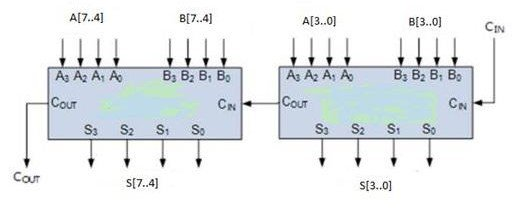Adders are used basically for performing calculations like arithmetic addition in digital calculators and they are also used in devices that works on the increment or arithmetic processes.

### CD4560 Proteus Example

This adder can perform addition of two 4-bit numbers. In decimal 4-bit number is equivalent to 0-15.  Therefore, it can perform addition upto 0-14. For example, Input A = 8 and Input B= 9, Carry out signal shows, adder overflow.

In this figure, we can see that we give both binary inputs as 00001. CD4560 gives output 0010 which is equal to decimal 2.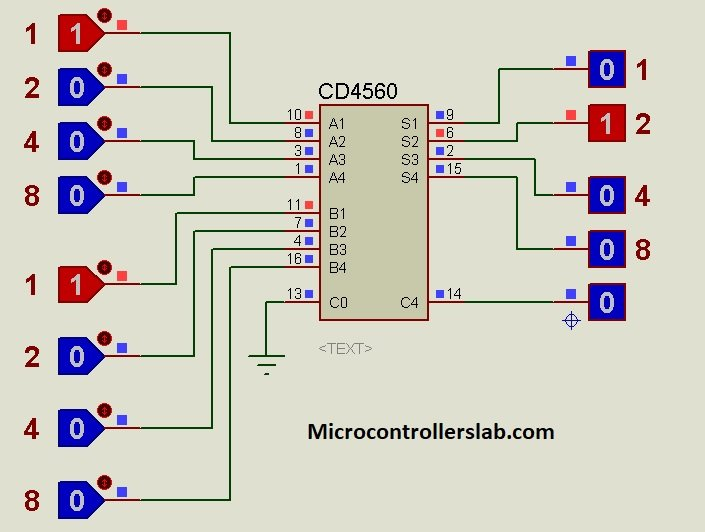Similarly, in this proteus simulation, we provide both input in decimal 7 and the output should be 14 or 1110 in binary. As we can see a same binary output.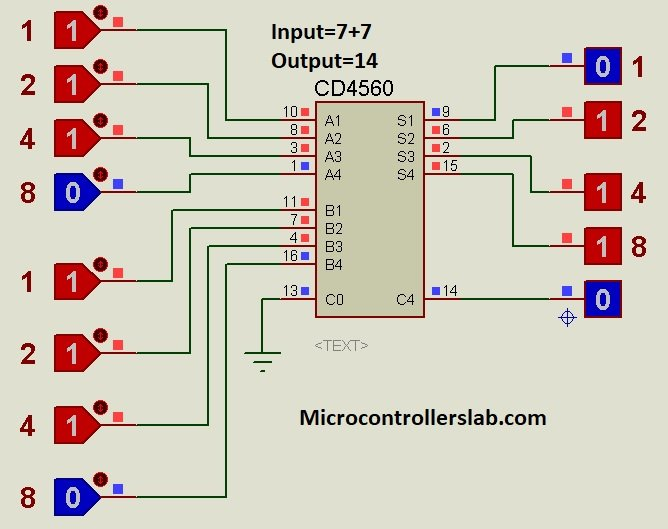We demonstrates carry out feature in this circuit diagram. Input A=15 and Input B = 7, Therefore, the ouput should be equal to 21. But this IC gives output between 0-15 only. However, if value increased this limit, carry out signal gives high output.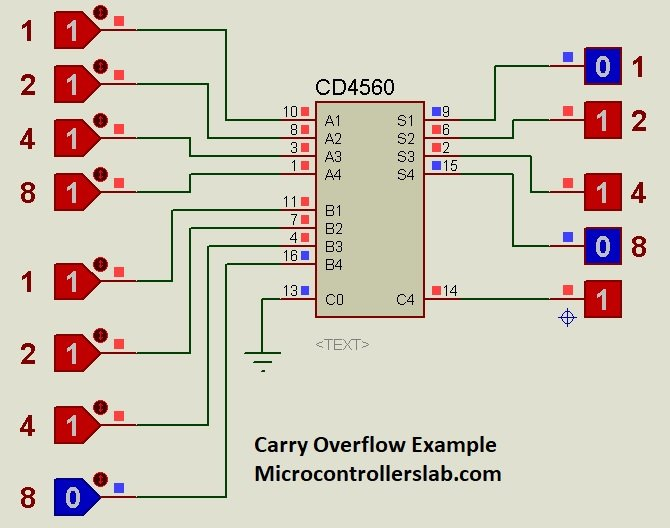## CD4560 Applications

CD4560 is mostly used in devices and applications performing calculations like addition.

• These ICs are wildly used in Arithmetic logic unit (ALU) to compute addition, Central Processing unit (CPU) and Graphics Processing unit (GPU) for graphics applications to reduce the circuit complexity.
• Adder is used in digital devices and electronic calculators for performing arithmetic operations like addition, subtraction, multiplication and division.
• They are also used in microcontrollers also for PC (program counter) and timers.
• In processors, they are used to calculate addresses, tables and similar operations
• It is also used in Digital signal processor and networking systems.